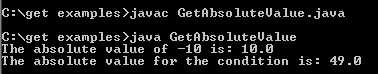Tutorials

# Get Absolute Value

In this example you will learn how to use the Absolute value is the only value of a number regardless of a prefixed plus or minus sign.

In this example you will learn how to use the Absolute value is the only value of a number regardless of a prefixed plus or minus sign.

# Get Absolute Value

Absolute value is the only value of a number regardless of a prefixed plus or minus sign. In the example given below we have used abs() method of class java.lang.Math that returns absolute value  of a given number as argument. We can use abs() method for double, float, int, long type values.

Here is the video tutorial of "How to calculate absolute value in Java?":

 public class GetAbsoluteValue {  public static void main(String args[]) throws Exception {   double value = Math.abs(-10);   double value1=Math.abs(1 - 50);   System.out.println("The absolute value of -10 is: "+value);   System.out.println("The absolute value for the condition is: "+value1);   } }

Output will be displayed as: